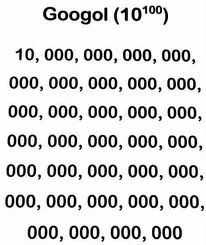# Really, really big numbers

### The realm of giants

How large is large? The question may seem circular, but to a mathematician, this is a good direction to explore. The answer depends on your perspective about how we measure largeness. Integers can be so massive they don’t correspond to things in the physical universe.  For example, a googol consists of 1 followed by 100 zeroes 10100.As the number of elementary particles of the universe is estimated to be around 1080, a googol staggers the imagination. And yes, the famous company resulted from a misspelling of this number.

However, integers form an infinite sequence: you can form a new one by adding one. Or multiply the number by itself, or take a power.  The number 10 to the power a googol is called googolplex, which is 10(10100). We soon run out of descriptive nomenclature to describe these large integers.

### Transfinite numbers

What about a number larger than all other numbers? Is this possible? Sort of.

Around the turn of the 20th century, Georg Cantor introduced a new kind of number called transfinite, which are all larger than the ordinary counting number or integers. If we consider all the non-negative integers as a single object or set, then we can consider that set itself to be a number. It is an infinite number, which is called ℵ0. Here “ℵ” is the first letter in Hebrew, and is pronounced “aleph”. The number ℵ0 (aleph zero) is the first infinite cardinal.

The cardinal ℵ0 has some strange properties. With a given positive integer, if you divide it or double it, you get a smaller or larger number. Not so with ℵ0. If you add it to itself, it doesn’t change:

0 + ℵ0 = ℵ0.

The same with multiplication:

00 = ℵ0.

The set of even integers is a strictly smaller set than the set of all positive integers, but it too has size ℵ0. So in a sense, dividing ℵ0 into smaller parts does not necessarily give a smaller number. These kind of observations seem puzzling at first, but they follow from simple facts about functions that we teach our second year mathematics undergraduates.

One can show that ℵ0 is the cardinality of not only the non-negative integers, but also of the set of all integers (positive and negative), and the set of rational numbers (or fractions). A simple test: if you can list an infinite set (more precisely, find a one-to-one function to the positive integers), then it has cardinality ℵ0.

The following diagram shows how to list all the rational numbers; the circled ones are “new” fractions in the ever expanding list. For example, we consider 2/2 the same as 1. You list all the distinct rationals by following the zig-zag arrow, starting with 1/1 = 1.By the way, don’t confuse ℵ0 with the symbol ∞.  The latter has no formal meaning as a number. Rather ∞ is used as notation in things like limits found in Calculus. Sorry Mr. Dali!

### Large cardinals

Like any good story, this is just the beginning: the infinite cardinals only just start at ℵ0. There is an infinite sequence of cardinals, each strictly bigger than the one before it:

0 < ℵ1 < ℵ2 < …

We even obtain much larger cardinals like ℵ0 that results by continuing the above process ℵ0-many times.

Things get even stranger when we consider exponentiation. The number 20 is strictly bigger than ℵ0.  The cardinal 20 is sometimes called the continuum, as it is the size of the real number system. The number of reals is much larger than the number of integers!  The famous Continuum hypothesis states that ℵ1 = 20, but whether this is true or not depends on the basic axioms we take for our mathematics, following from the foundational work of Gödel and Cohen.

The mathematics of cardinals quickly gets into deep waters and is not fully understood. There are esoteric-seeming large cardinals, which exist only under special hypotheses in the realm of set theory. So-called large cardinal theory focuses on describing and classifying such cardinals, with esoteric sounding names such as Woodin, strongly Ramsey, and supercompact cardinals.

We therefore have an infinite hierarchy of infinite numbers, without end. The collection of all cardinals together is so large it is not a set in the usual sense. This collection is what is called a proper class. We can’t talk about the cardinality of a proper class, as it bigger than every cardinality.

### The very small: Conway’s surreal numbers

We have discussed very large numbers, but what about the very small? Real numbers like 1/10100 are easy to write down, but incomprehensibly small. A Planck length is 1.6 x 10-35 meters, and is reserved for the smallest objects in the universe. Physicists speculate that a string has size a Planck length. Strings, however, have yet to be observed.

John Conway discovered the surreal numbers that include infinitesimal numbers, or numbers greater than 0 but smaller than any fraction. These unusual numbers correspond to values of certain combinatorial games, and include infinite cardinals, infinitesimals, and exotic numbers like *.While surreal explain properties of certain games, to date, there are no applications of the surreal numbers in the sciences. But this will almost certainly change as science advances. Like Hamilton’s quaternions, which are now routinely applied in physics and engineering, the surreal numbers are just so beautiful that they simply must be applicable.